# snssde1d()

Assume that we want to describe the following SDE:

Itô form3:

$\begin{equation}\label{eq:05} dX_{t} = \frac{1}{2}\theta^{2} X_{t} dt + \theta X_{t} dW_{t},\qquad X_{0}=x_{0} > 0 \end{equation}$ Stratonovich form: $\begin{equation}\label{eq:06} dX_{t} = \frac{1}{2}\theta^{2} X_{t} dt +\theta X_{t} \circ dW_{t},\qquad X_{0}=x_{0} > 0 \end{equation}$

In the above $$f(t,x)=\frac{1}{2}\theta^{2} x$$ and $$g(t,x)= \theta x$$ ($$\theta > 0$$), $$W_{t}$$ is a standard Wiener process. To simulate this models using snssde1d() function we need to specify:

• The drift and diffusion coefficients as R expressions that depend on the state variable x and time variable t.
• The number of simulation steps N=1000 (by default: N=1000).
• The number of the solution trajectories to be simulated by M=1000 (by default: M=1).
• The initial conditions t0=0, x0=10 and end time T=1 (by default: t0=0, x0=0 and T=1).
• The integration step size Dt=0.001 (by default: Dt=(T-t0)/N).
• The choice of process types by the argument type="ito" for Ito or type="str" for Stratonovich (by default type="ito").
• The numerical method to be used by method (by default method="euler").
R> theta = 0.5
R> f <- expression( (0.5*theta^2*x) )
R> g <- expression( theta*x )
R> mod1 <- snssde1d(drift=f,diffusion=g,x0=10,M=1000,type="ito") # Using Itô
R> mod2 <- snssde1d(drift=f,diffusion=g,x0=10,M=1000,type="str") # Using Stratonovich
R> mod1
Itô Sde 1D:
| dX(t) = (0.5 * theta^2 * X(t)) * dt + theta * X(t) * dW(t)
Method:
| Euler scheme with order 0.5
Summary:
| Size of process   | N  = 1001.
| Number of simulation  | M  = 1000.
| Initial value     | x0 = 10.
| Time of process   | t in [0,1].
| Discretization    | Dt = 0.001.
R> mod2
Stratonovich Sde 1D:
| dX(t) = (0.5 * theta^2 * X(t)) * dt + theta * X(t) o dW(t)
Method:
| Euler scheme with order 0.5
Summary:
| Size of process   | N  = 1001.
| Number of simulation  | M  = 1000.
| Initial value     | x0 = 10.
| Time of process   | t in [0,1].
| Discretization    | Dt = 0.001.

Using Monte-Carlo simulations, the following statistical measures (S3 method) for class snssde1d() can be approximated for the $$X_{t}$$ process at any time $$t$$:

• The expected value $$\text{E}(X_{t})$$ at time $$t$$, using the command mean.
• The variance $$\text{Var}(X_{t})$$ at time $$t$$, using the command moment with order=2 and center=TRUE.
• The median $$\text{Med}(X_{t})$$ at time $$t$$, using the command Median.
• The mode $$\text{Mod}(X_{t})$$ at time $$t$$, using the command Mode.
• The quartile of $$X_{t}$$ at time $$t$$, using the command quantile.
• The maximum and minimum of $$X_{t}$$ at time $$t$$, using the command min and max.
• The skewness and the kurtosis of $$X_{t}$$ at time $$t$$, using the command skewness and kurtosis.
• The coefficient of variation (relative variability) of $$X_{t}$$ at time $$t$$, using the command cv.
• The central moments up to order $$p$$ of $$X_{t}$$ at time $$t$$, using the command moment.
• The empirical $$\alpha \%$$ confidence interval of expected value $$\text{E}(X_{t})$$ at time $$t$$ (from the $$2.5th$$ to the $$97.5th$$ percentile), using the command bconfint.
• The result summaries of the results of Monte-Carlo simulation at time $$t$$, using the command summary.

The following statistical measures (S3 method) for class snssde1d() can be approximated for the $$X_{t}$$ process at any time $$t$$, for example at=1:

R> s = 1
R> mean(mod1, at = s)
 11.309
R> moment(mod1, at = s , center = TRUE , order = 2) ## variance
 36.973
R> Median(mod1, at = s)
 9.9856
R> Mode(mod1, at =s)
 9.0918
R> quantile(mod1 , at = s)
     0%     25%     50%     75%    100%
1.8793  7.1417  9.9856 13.9511 51.1379 
R> kurtosis(mod1 , at = s)
 7.4572
R> skewness(mod1 , at = s)
 1.6261
R> cv(mod1 , at = s )
 0.53796
R> min(mod1 , at = s)
 1.8793
R> max(mod1 , at = s)
 51.138
R> moment(mod1, at = s , center= TRUE , order = 4)
 10215
R> moment(mod1, at = s , center= FALSE , order = 4)
 71501

The summary of the results of mod1 and mod2 at time $$t=1$$ of class snssde1d() is given by:

R> summary(mod1, at = 1)

Monte-Carlo Statistics for X(t) at time t = 1

Mean                  11.30864
Variance              37.01033
Median                 9.98564
Mode                   9.09179
First quartile         7.14169
Third quartile        13.95111
Minimum                1.87935
Maximum               51.13790
Skewness               1.62614
Kurtosis               7.45721
Coef-variation         0.53796
3th-order moment     366.13591
4th-order moment   10214.62053
5th-order moment  261731.91301
6th-order moment 8119638.43329
R> summary(mod2, at = 1)

Monte-Carlo Statistics for X(t) at time t = 1

Mean                  10.12993
Variance              30.58754
Median                 8.78553
Mode                   6.63369
First quartile         6.21806
Third quartile        12.65557
Minimum                2.07253
Maximum               51.83341
Skewness               1.68558
Kurtosis               8.30989
Coef-variation         0.54597
3th-order moment     285.14567
4th-order moment    7774.71311
5th-order moment  217522.03698
6th-order moment 7490738.91929

Hence we can just make use of the rsde1d() function to build our random number generator for the conditional density of the $$X_{t}|X_{0}$$ ($$X_{t}^{\text{mod1}}| X_{0}$$ and $$X_{t}^{\text{mod2}}|X_{0}$$) at time $$t = 1$$.

R> x1 <- rsde1d(object = mod1, at = 1)  # X(t=1) | X(0)=x0 (Itô SDE)
R> x2 <- rsde1d(object = mod2, at = 1)  # X(t=1) | X(0)=x0 (Stratonovich SDE)
R> head(x1,n=10)
   7.1408 12.7503 24.7303 12.5644 13.0793  6.4470  8.7430  6.1797
 11.7056 10.1324
R> head(x2,n=10)
  18.2654  7.2851  3.8816  6.8366 17.5960  8.4661  5.1722 11.7376
 17.8945  7.5208
R> summary(data.frame(x1,x2))
       x1              x2
Min.   : 1.88   Min.   : 2.07
1st Qu.: 7.14   1st Qu.: 6.22
Median : 9.99   Median : 8.79
Mean   :11.31   Mean   :10.13
3rd Qu.:13.95   3rd Qu.:12.66
Max.   :51.14   Max.   :51.83  

The function dsde1d() can be used to show the Approximate transitional density for $$X_{t}|X_{0}$$ at time $$t-s=1$$ with log-normal curves:

R> mu1 = log(10); sigma1= sqrt(theta^2)  # log mean and log variance for mod1
R> mu2 = log(10)-0.5*theta^2 ; sigma2 = sqrt(theta^2) # log mean and log variance for mod2
R> AppdensI <- dsde1d(mod1, at = 1)
R> AppdensS <- dsde1d(mod2, at = 1)
R> plot(AppdensI , dens = function(x) dlnorm(x,meanlog=mu1,sdlog = sigma1))
R> plot(AppdensS , dens = function(x) dlnorm(x,meanlog=mu2,sdlog = sigma2))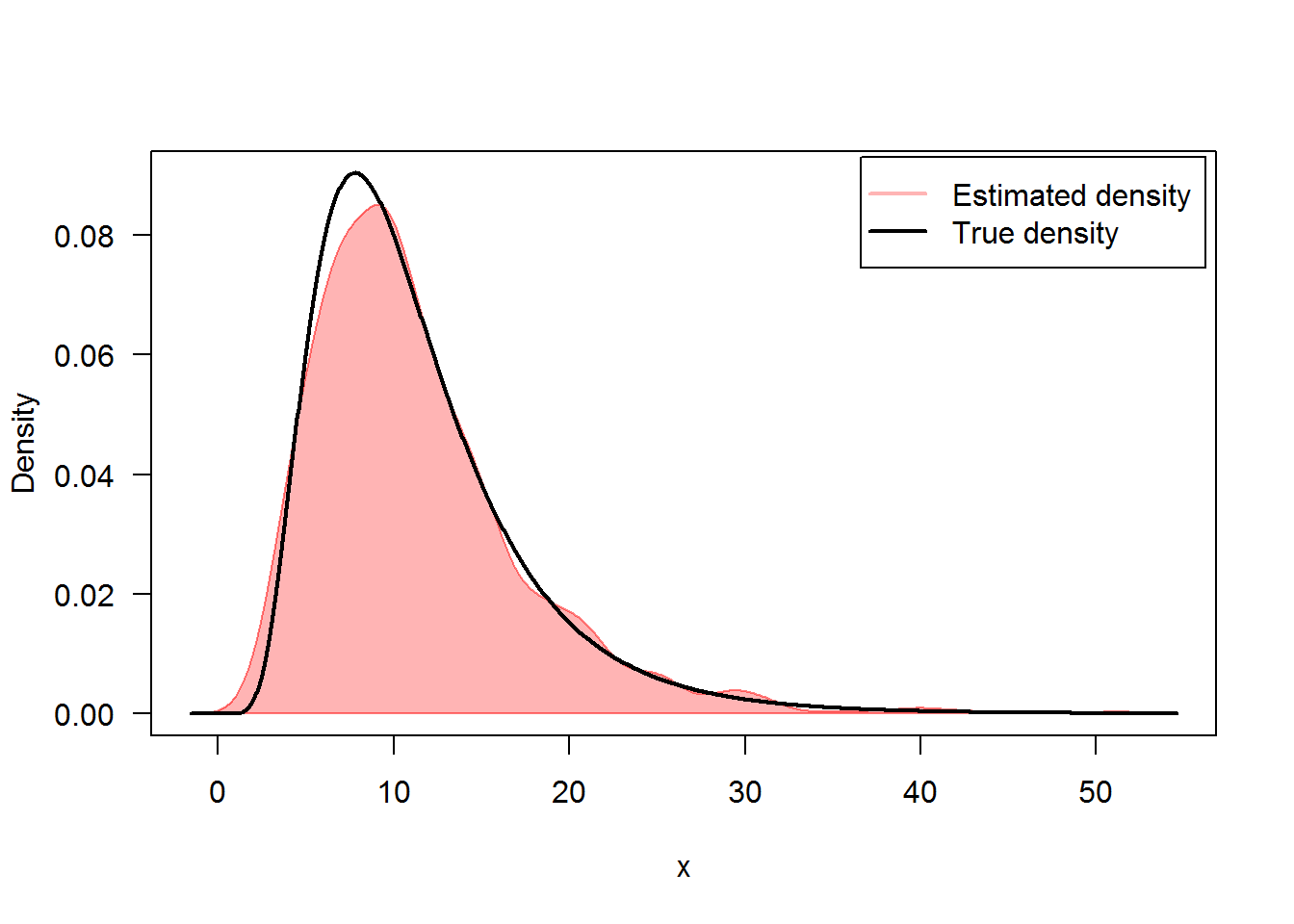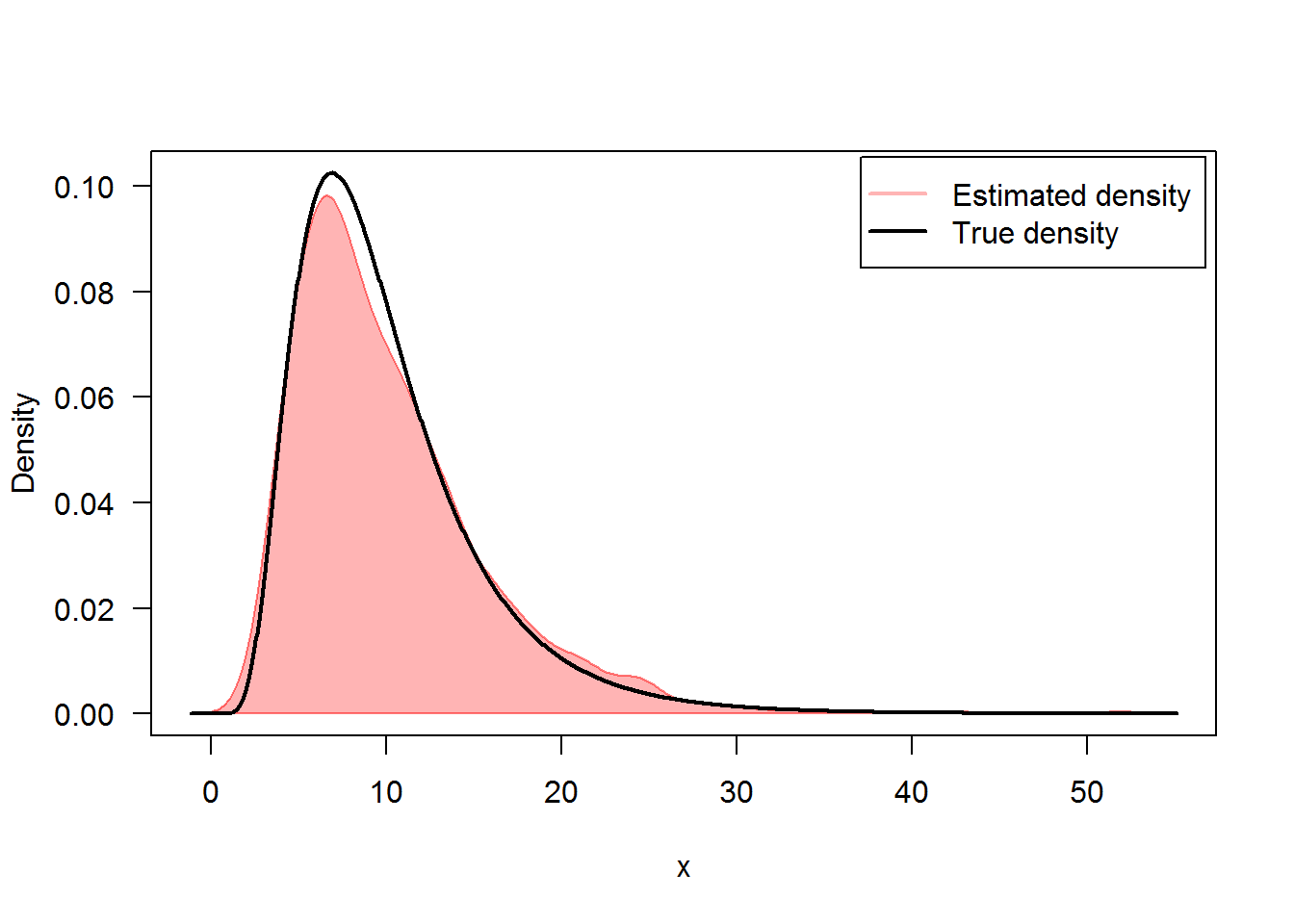In Figure 2, we present the flow of trajectories, the mean path (red lines) of solution of and , with their empirical $$95\%$$ confidence bands, that is to say from the $$2.5th$$ to the $$97.5th$$ percentile for each observation at time $$t$$ (blue lines):

R> ## Itô
R> plot(mod1,ylab=expression(X^mod1))
R> lines(time(mod1),apply(mod1$X,1,mean),col=2,lwd=2) R> lines(time(mod1),apply(mod1$X,1,bconfint,level=0.95)[1,],col=4,lwd=2)
R> lines(time(mod1),apply(mod1$X,1,bconfint,level=0.95)[2,],col=4,lwd=2) R> legend("topleft",c("mean path",paste("bound of", 95,"% confidence")),inset = .01,col=c(2,4),lwd=2,cex=0.8) R> ## Stratonovich R> plot(mod2,ylab=expression(X^mod2)) R> lines(time(mod2),apply(mod2$X,1,mean),col=2,lwd=2)
R> lines(time(mod2),apply(mod2$X,1,bconfint,level=0.95)[1,],col=4,lwd=2) R> lines(time(mod2),apply(mod2$X,1,bconfint,level=0.95)[2,],col=4,lwd=2)
R> legend("topleft",c("mean path",paste("bound of",95,"% confidence")),col=c(2,4),inset =.01,lwd=2,cex=0.8)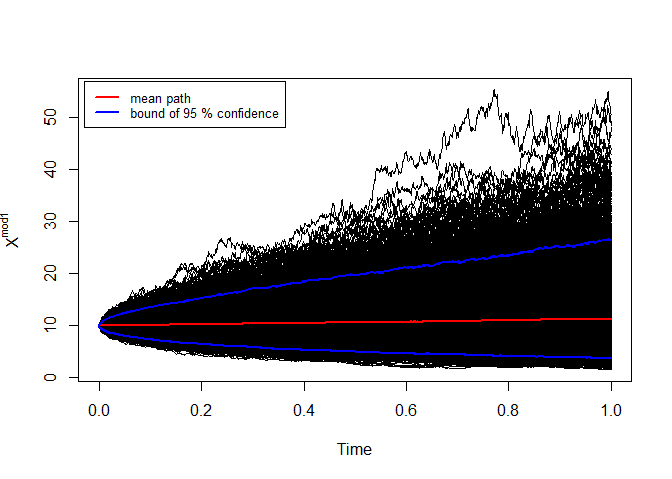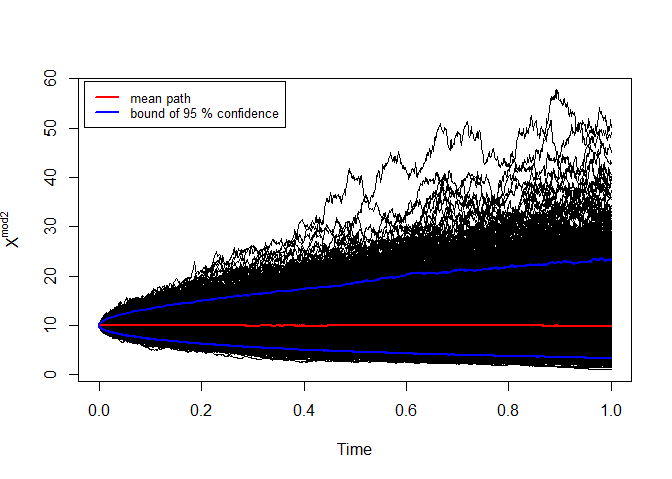mod1: Itô and mod2: Stratonovich

# snssde2d()

The following $$2$$-dimensional SDE’s with a vector of drift and a diagonal matrix of diffusion coefficients:

Itô form: $\begin{equation}\label{eq:09} \begin{cases} dX_t = f_{x}(t,X_{t},Y_{t}) dt + g_{x}(t,X_{t},Y_{t}) dW_{1,t}\\ dY_t = f_{y}(t,X_{t},Y_{t}) dt + g_{y}(t,X_{t},Y_{t}) dW_{2,t} \end{cases} \end{equation}$ Stratonovich form: $\begin{equation}\label{eq:10} \begin{cases} dX_t = f_{x}(t,X_{t},Y_{t}) dt + g_{x}(t,X_{t},Y_{t}) \circ dW_{1,t}\\ dY_t = f_{y}(t,X_{t},Y_{t}) dt + g_{y}(t,X_{t},Y_{t}) \circ dW_{2,t} \end{cases} \end{equation}$

$$W_{1,t}$$ and $$W_{2,t}$$ is a two independent standard Wiener process. To simulate $$2d$$ models using snssde2d() function we need to specify:

• The drift (2d) and diffusion (2d) coefficients as R expressions that depend on the state variable x, y and time variable t.
• The number of simulation steps N (default: N=1000).
• The number of the solution trajectories to be simulated by M (default: M=1).
• The initial conditions t0, x0 and end time T (default: t0=0, x0=c(0,0) and T=1).
• The integration step size Dt (default: Dt=(T-t0)/N).
• The choice of process types by the argument type="ito" for Ito or type="str" for Stratonovich (default type="ito").
• The numerical method to be used by method (default method="euler").

## Ornstein-Uhlenbeck process and its integral

The Ornstein-Uhlenbeck (OU) process has a long history in physics. Introduced in essence by Langevin in his famous 1908 paper on Brownian motion, the process received a more thorough mathematical examination several decades later by Uhlenbeck and Ornstein (1930). The OU process is understood here to be the univariate continuous Markov process $$X_t$$. In mathematical terms, the equation is written as an Ito equation: $\begin{equation}\label{eq016} dX_t = -\frac{1}{\mu} X_t dt + \sqrt{\sigma} dW_t,\quad X_{0}=x_{0} \end{equation}$ In these equations, $$\mu$$ and $$\sigma$$ are positive constants called, respectively, the relaxation time and the diffusion constant. The time integral of the OU process $$X_t$$ (or indeed of any process $$X_t$$) is defined to be the process $$Y_t$$ that satisfies: $\begin{equation}\label{eq017} Y_{t} = Y_{0}+\int X_{t} dt \Leftrightarrow dY_t = X_{t} dt ,\quad Y_{0}=y_{0} \end{equation}$ $$Y_t$$ is not itself a Markov process; however, $$X_t$$ and $$Y_t$$ together comprise a bivariate continuous Markov process. We wish to find the solutions $$X_t$$ and $$Y_t$$ to the coupled time-evolution equations: $\begin{equation}\label{eq018} \begin{cases} dX_t = -\frac{1}{\mu} X_t dt + \sqrt{\sigma} dW_t\\ dY_t = X_{t} dt \end{cases} \end{equation}$

We simulate a flow of $$1000$$ trajectories of $$(X_{t},Y_{t})$$, with integration step size $$\Delta t = 0.01$$, and using second Milstein method.

R> x0=5;y0=0
R> mu=3;sigma=0.5
R> fx <- expression(-(x/mu),x)
R> gx <- expression(sqrt(sigma),0)
R> mod2d <- snssde2d(drift=fx,diffusion=gx,Dt=0.01,M=1000,x0=c(x0,y0),method="smilstein")
R> mod2d
Itô Sde 2D:
| dX(t) = -(X(t)/mu) * dt + sqrt(sigma) * dW1(t)
| dY(t) = X(t) * dt + 0 * dW2(t)
Method:
| Second-order Milstein scheme
Summary:
| Size of process   | N  = 1001.
| Number of simulation  | M  = 1000.
| Initial values    | (x0,y0) = (5,0).
| Time of process   | t in [0,10].
| Discretization    | Dt = 0.01.

The following statistical measures (S3 method) for class snssde2d() can be approximated for the $$(X_{t},Y_{t})$$ process at any time $$t$$, for example at=5:

R> s = 5
R> mean(mod2d, at = s)
  0.98131 12.24217
R> moment(mod2d, at = s , center = TRUE , order = 2) ## variance
 0.74467 7.06026
R> Median(mod2d, at = s)
  1.0062 12.2806
R> Mode(mod2d, at = s)
  1.2937 12.0354
R> quantile(mod2d , at = s)
$x 0% 25% 50% 75% 100% -1.72370 0.38862 1.00617 1.59448 3.68060$y
0%     25%     50%     75%    100%
1.6918 10.3693 12.2806 14.1281 21.6861 
R> kurtosis(mod2d , at = s)
 2.8482 2.9520
R> skewness(mod2d , at = s)
 -0.11694 -0.12734
R> cv(mod2d , at = s )
 0.87982 0.21715
R> min(mod2d , at = s)
 -1.7237  1.6918
R> max(mod2d , at = s)
  3.6806 21.6861
R> moment(mod2d, at = s , center= TRUE , order = 4)
   1.5826 147.4459
R> moment(mod2d, at = s , center= FALSE , order = 4)
     6.5171 28840.3253

The summary of the results of mod2d at time $$t=5$$ of class snssde2d() is given by:

R> summary(mod2d, at = s)

Monte-Carlo Statistics for (X(t),Y(t)) at time t = 5
X          Y
Mean              0.98131   12.24217
Variance          0.74542    7.06733
Median            1.00617   12.28057
Mode              1.29371   12.03535
First quartile    0.38862   10.36932
Third quartile    1.59448   14.12811
Minimum          -1.72370    1.69180
Maximum           3.68060   21.68613
Skewness         -0.11694   -0.12734
Kurtosis          2.84818    2.95204
Coef-variation    0.87982    0.21715
3th-order moment -0.07526   -2.39241
4th-order moment  1.58259  147.44592
5th-order moment -0.35759 -166.53893
6th-order moment  5.40569 5739.27245

For plotting (back in time) using the command plot, the results of the simulation are shown in Figure 3.

R> plot(mod2d)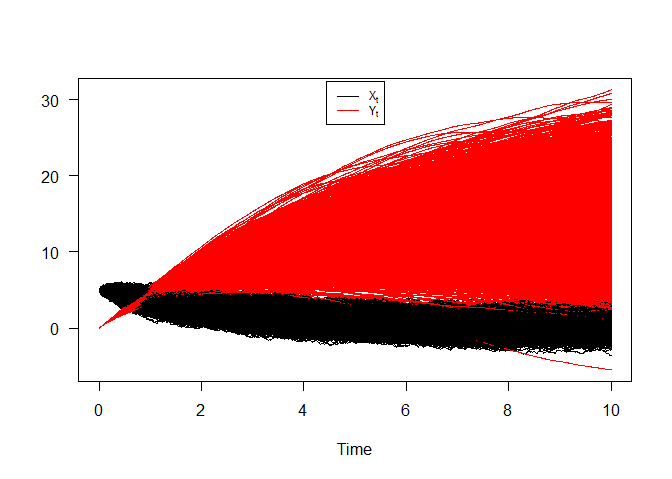Ornstein-Uhlenbeck process and its integral

Take note of the well known result, which can be derived from either this equations. That for any $$t > 0$$ the OU process $$X_t$$ and its integral $$Y_t$$ will be the normal distribution with mean and variance given by: $\begin{cases} \text{E}(X_{t}) =x_{0} e^{-t/\mu} &\text{and}\quad\text{Var}(X_{t})=\frac{\sigma \mu}{2} \left (1-e^{-2t/\mu}\right )\\ \text{E}(Y_{t}) = y_{0}+x_{0}\mu \left (1-e^{-t/\mu}\right ) &\text{and}\quad\text{Var}(Y_{t})=\sigma\mu^{3}\left (\frac{t}{\mu}-2\left (1-e^{-t/\mu}\right )+\frac{1}{2}\left (1-e^{-2t/\mu}\right )\right ) \end{cases}$

Hence we can just make use of the rsde2d() function to build our random number for $$(X_{t},Y_{t})$$ at time $$t = 10$$.

R> out <- rsde2d(object = mod2d, at = 10)
R> head(out,n=10)
           x       y
1   0.605260 17.4761
2   0.038043 10.0443
3  -1.044941 14.9122
4   0.558719 13.9970
5  -0.133361 13.7066
6  -1.414328  8.8593
7   0.107658 10.6190
8   0.662425 18.4829
9   0.729330 10.1667
10 -0.188384 17.4795
R> summary(out)
       x                y
Min.   :-3.287   Min.   :-3.08
1st Qu.:-0.399   1st Qu.:11.21
Median : 0.226   Median :14.91
Mean   : 0.212   Mean   :14.76
3rd Qu.: 0.822   3rd Qu.:18.28
Max.   : 2.954   Max.   :28.79  
R> cov(out)
        x       y
x 0.81247  2.3758
y 2.37577 26.8571

Figure 4, show simulation results for moments of system :

R> mx <- apply(mod2d$X,1,mean) R> my <- apply(mod2d$Y,1,mean)
R> Sx <- apply(mod2d$X,1,var) R> Sy <- apply(mod2d$Y,1,var)
R> Cxy <- sapply(1:1001,function(i) cov(mod2d$X[i,],mod2d$Y[i,]))
R> out_b <- data.frame(mx,my,Sx,Sy,Cxy)
R> matplot(time(mod2d), out_b, type = "l", xlab = "time", ylab = "",col=2:6,lwd=2,lty=2:6,las=1)
R> legend("topleft",c(expression(hat(E)(X[t]),hat(E)(Y[t]),hat(Var)(X[t]),hat(Var)(Y[t]),hat(Cov)(X[t],Y[t]))),inset = .05,col=2:6,lty=2:6,lwd=2,cex=0.9)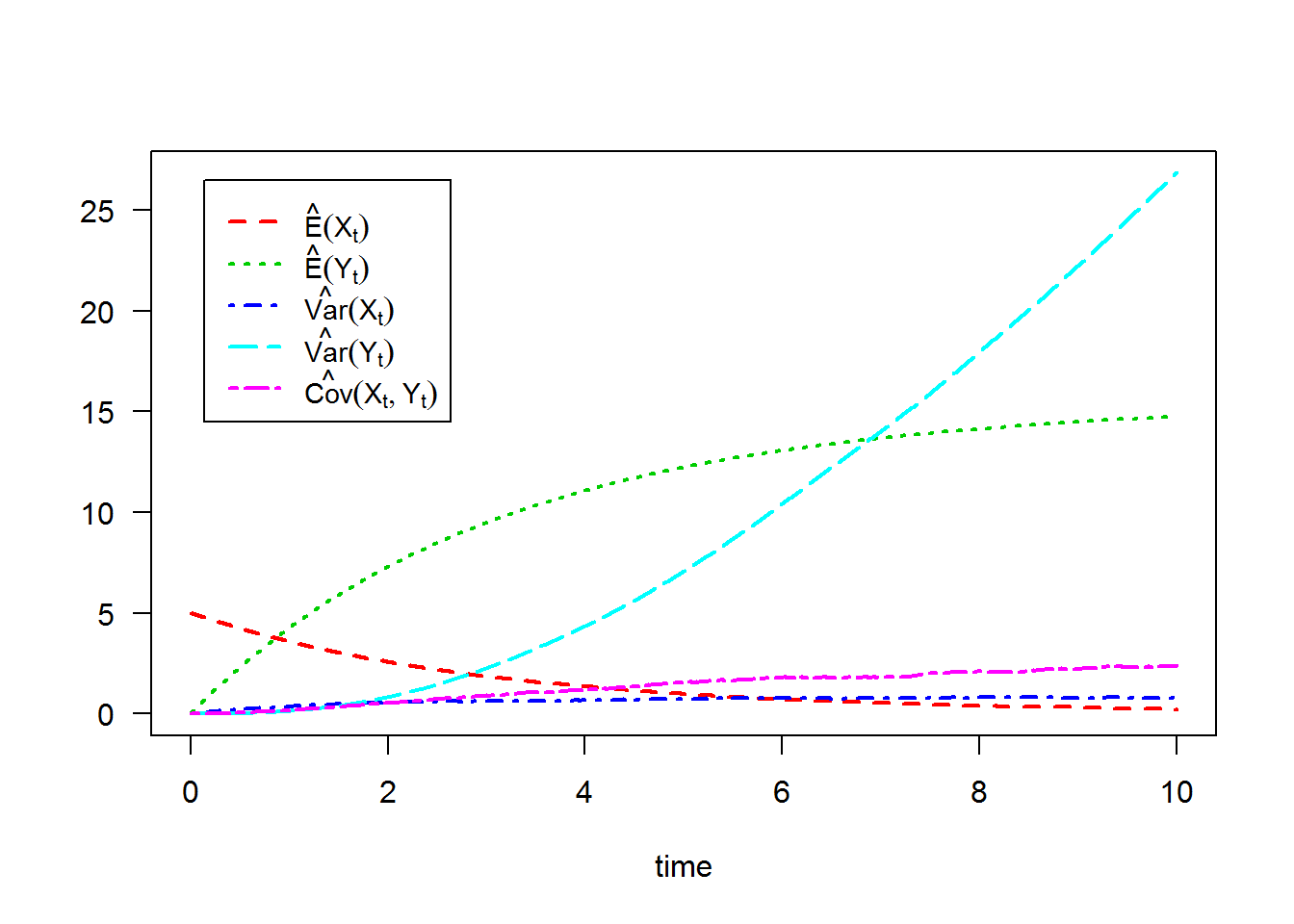For each SDE type and for each numerical scheme, the density of $$X_t$$ and $$Y_t$$ at time $$t=10$$ are reported using dsde2d() function, see e.g. Figure 5: the marginal density of $$X_t$$ and $$Y_t$$ at time $$t=10$$.

R> denM <- dsde2d(mod2d,pdf="M",at =10)
R> denM

Marginal density of X(t-t0)|X(t0)=5 at time t = 10

Data: x (1000 obs.);    Bandwidth 'bw' = 0.2038

x                f(x)
Min.   :-3.8978   Min.   :0.00002
1st Qu.:-2.0319   1st Qu.:0.00391
Median :-0.1660   Median :0.05077
Mean   :-0.1660   Mean   :0.13385
3rd Qu.: 1.6998   3rd Qu.:0.25711
Max.   : 3.5657   Max.   :0.42899

Marginal density of Y(t-t0)|Y(t0)=0 at time t = 10

Data: y (1000 obs.);    Bandwidth 'bw' = 1.172

y               f(y)
Min.   :-6.599   Min.   :0.000004
1st Qu.: 3.127   1st Qu.:0.001576
Median :12.852   Median :0.012469
Mean   :12.852   Mean   :0.025680
3rd Qu.:22.578   3rd Qu.:0.051069
Max.   :32.303   Max.   :0.073983  
R> plot(denM, main="Marginal Density")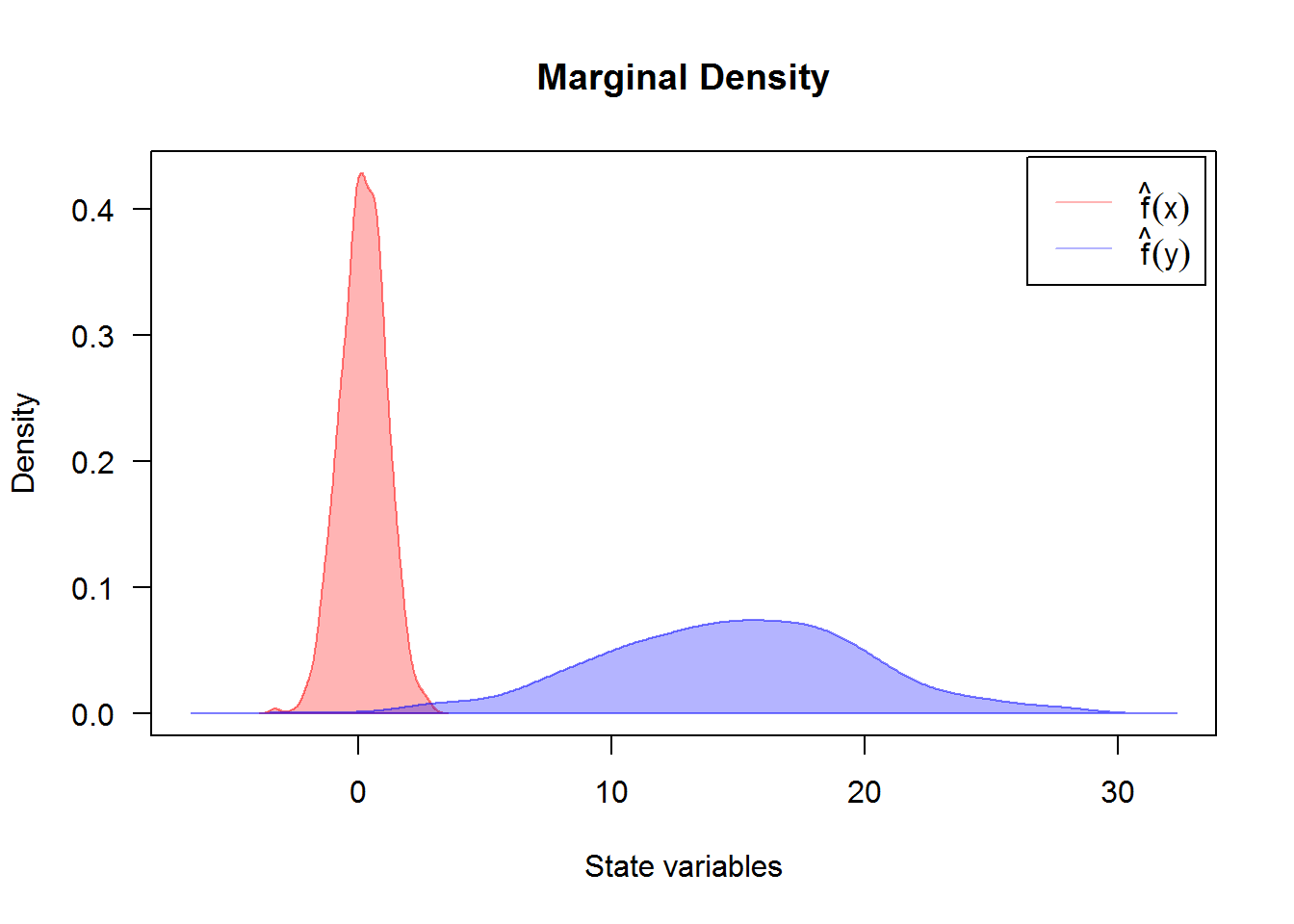Created using dsde2d() plotted in (x, y)-space with dim = 2. A contour and image plot of density obtained from a realization of system $$(X_{t},Y_{t})$$ at time t=10.

R> denJ <- dsde2d(mod2d, pdf="J", n=100,at =10)
R> denJ

Joint density of (X(t-t0),Y(t-t0)|X(t0)=5,Y(t0)=0) at time t = 10

Data: (x,y) (2 x 1000 obs.);

x                 y               f(x,y)
Min.   :-3.2865   Min.   :-3.0842   Min.   :0.000000
1st Qu.:-1.7262   1st Qu.: 4.8840   1st Qu.:0.000028
Median :-0.1660   Median :12.8522   Median :0.000976
Mean   :-0.1660   Mean   :12.8522   Mean   :0.004902
3rd Qu.: 1.3942   3rd Qu.:20.8204   3rd Qu.:0.005444
Max.   : 2.9544   Max.   :28.7886   Max.   :0.035428  
R> plot(denJ,display="contour",main="Bivariate Transition Density at time t=10")
R> plot(denJ,display="image",main="Bivariate Transition Density at time t=10")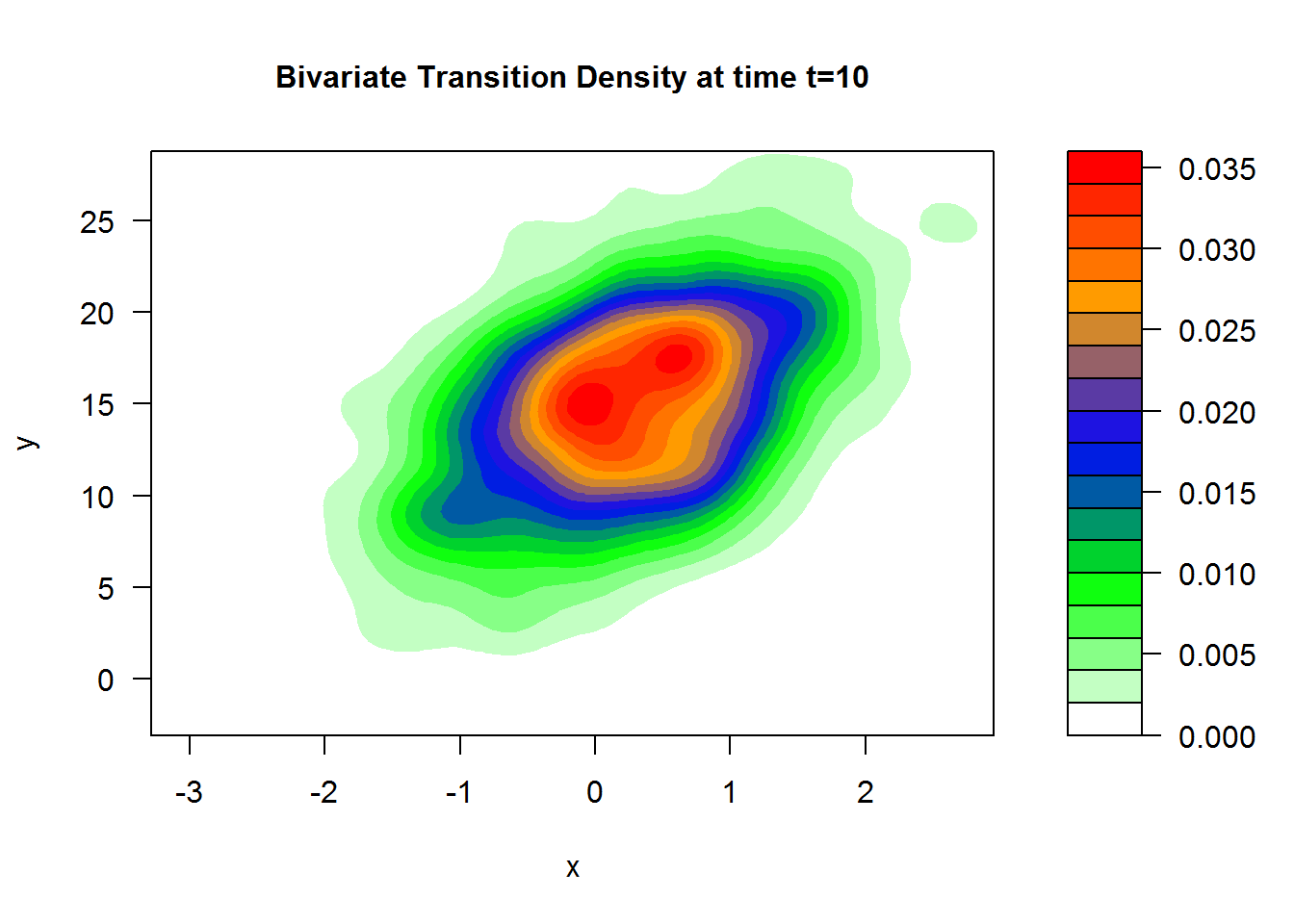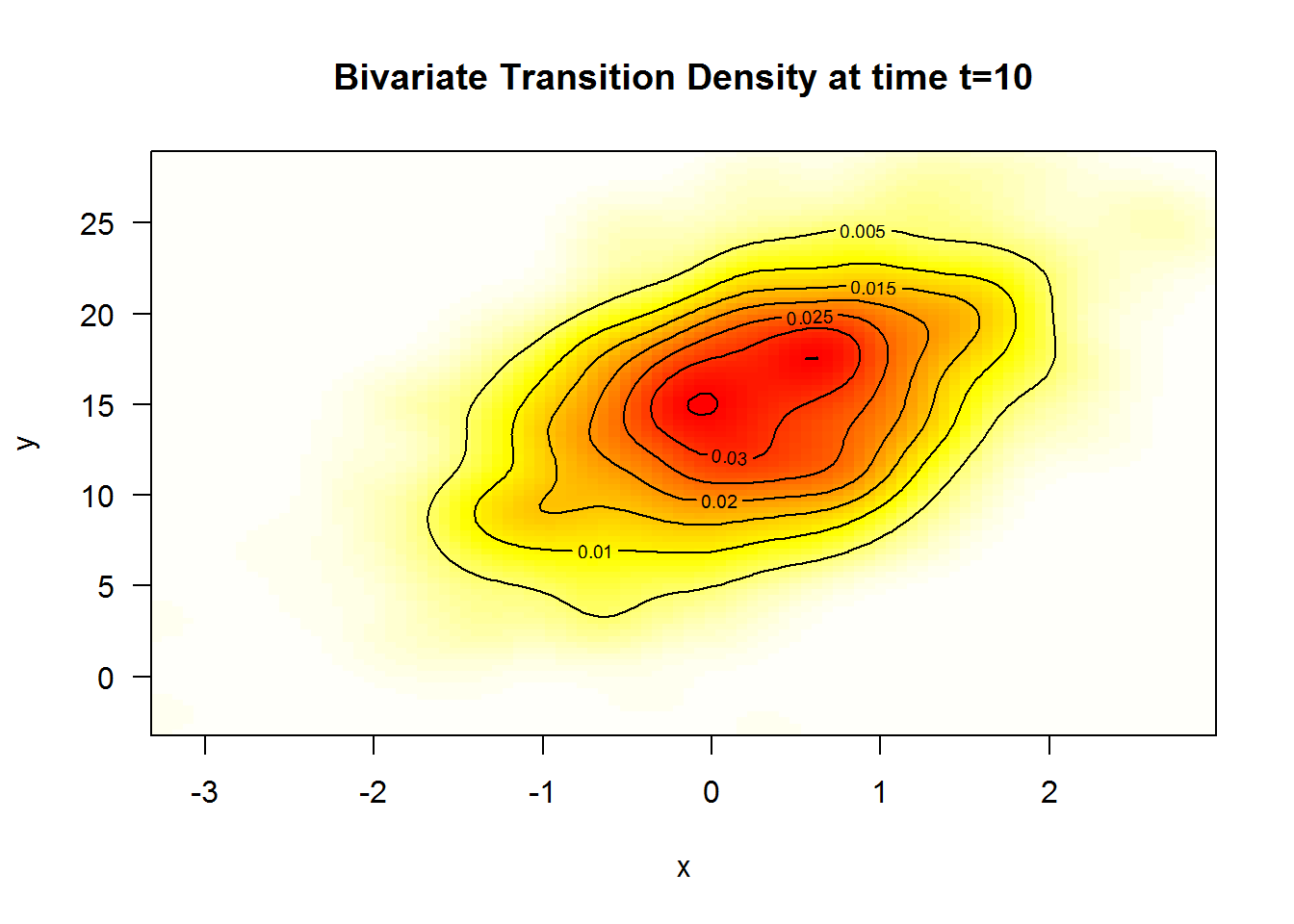A $$3$$D plot of the transition density at $$t=10$$ obtained with:

R> plot(denJ,main="Bivariate Transition Density at time t=10")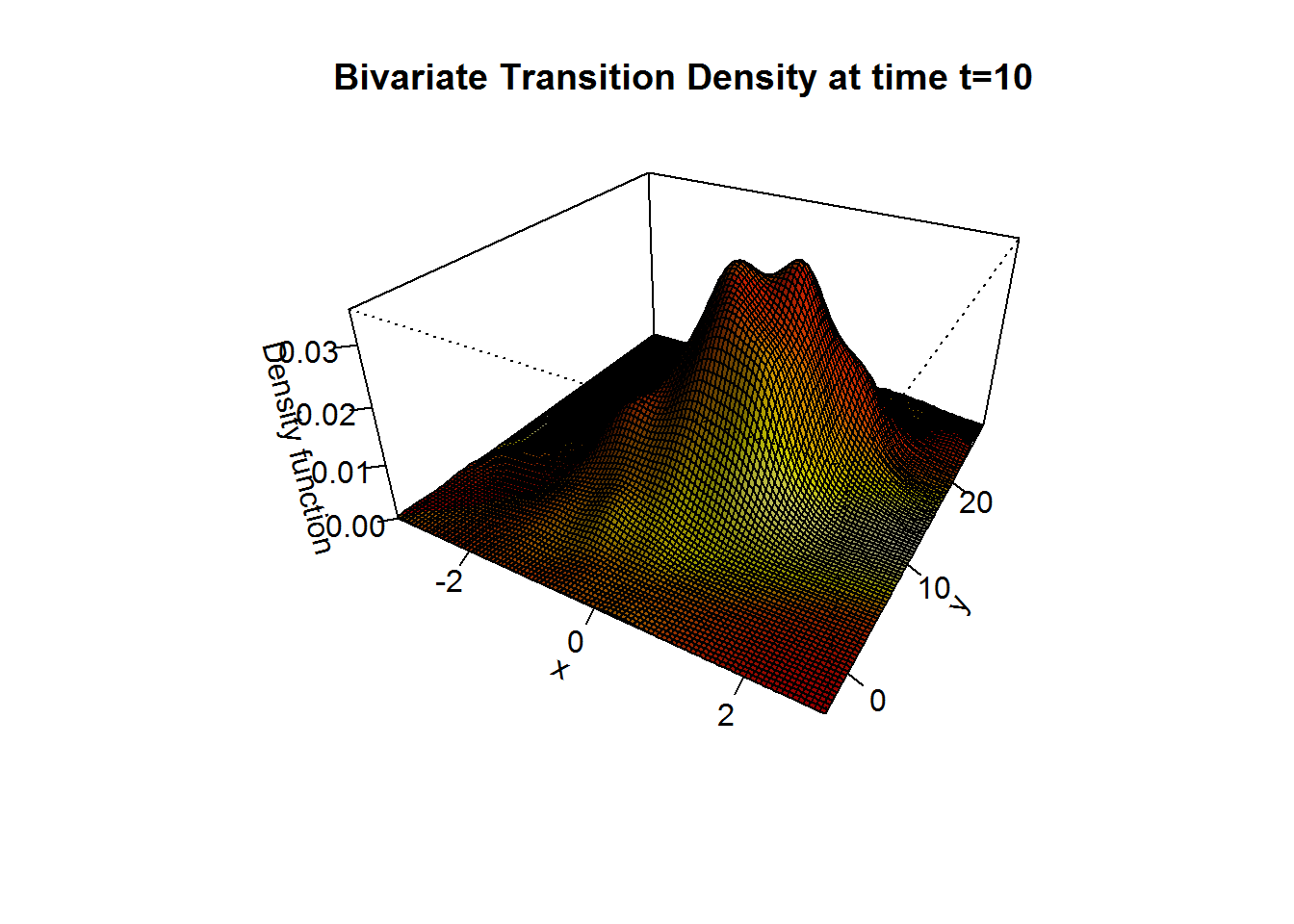We approximate the bivariate transition density over the set transition horizons $$t\in [1,10]$$ by $$\Delta t = 0.005$$ using the code:

R> for (i in seq(1,10,by=0.005)){
+ plot(dsde2d(mod2d, at = i,n=100),display="contour",main=paste0('Transition Density \n t = ',i))
+ }

## The stochastic Van-der-Pol equation

The Van der Pol (1922) equation is an ordinary differential equation that can be derived from the Rayleigh differential equation by differentiating and setting $$\dot{x}=y$$, see Naess and Hegstad (1994); Leung (1995) and for more complex dynamics in Van-der-Pol equation see Jing et al. (2006). It is an equation describing self-sustaining oscillations in which energy is fed into small oscillations and removed from large oscillations. This equation arises in the study of circuits containing vacuum tubes and is given by: $\begin{equation}\label{eq:12} \ddot{X}-\mu (1-X^{2}) \dot{X} + X = 0 \end{equation}$ where $$x$$ is the position coordinate (which is a function of the time $$t$$), and $$\mu$$ is a scalar parameter indicating the nonlinearity and the strength of the damping, to simulate the deterministic equation see Grayling (2014) for more details. Consider stochastic perturbations of the Van-der-Pol equation, and random excitation force of such systems by White noise $$\xi_{t}$$, with delta-type correlation function $$\text{E}(\xi_{t}\xi_{t+h})=2\sigma \delta (h)$$ $\begin{equation}\label{eq:13} \ddot{X}-\mu (1-X^{2}) \dot{X} + X = \xi_{t}, \end{equation}$ where $$\mu > 0$$ . It’s solution cannot be obtained in terms of elementary functions, even in the phase plane. The White noise $$\xi_{t}$$ is formally derivative of the Wiener process $$W_{t}$$. The representation of a system of two first order equations follows the same idea as in the deterministic case by letting $$\dot{x}=y$$, from physical equation we get the above system: $\begin{equation}\label{eq:14} \begin{cases} \dot{X} = Y \\ \dot{Y} = \mu \left(1-X^{2}\right) Y - X + \xi_{t} \end{cases} \end{equation}$ The system can mathematically explain by a Stratonovitch equations: $\begin{equation}\label{eq:15} \begin{cases} dX_{t} = Y_{t} dt \\ dY_{t} = \left(\mu (1-X^{2}_{t}) Y_{t} - X_{t}\right) dt + 2 \sigma \circ dW_{2,t} \end{cases} \end{equation}$

Implemente in R as follows, with integration step size $$\Delta t = 0.01$$ and using stochastic Runge-Kutta methods 1-stage.

R> mu = 4; sigma=0.1
R> fx <- expression( y ,  (mu*( 1-x^2 )* y - x))
R> gx <- expression( 0 ,2*sigma)
R> mod2d <- snssde2d(drift=fx,diffusion=gx,N=10000,Dt=0.01,type="str",method="rk1")
R> mod2d
Stratonovich Sde 2D:
| dX(t) = Y(t) * dt + 0 o dW1(t)
| dY(t) = (mu * (1 - X(t)^2) * Y(t) - X(t)) * dt + 2 * sigma o dW2(t)
Method:
| Runge-Kutta method with order 1
Summary:
| Size of process   | N  = 10001.
| Number of simulation  | M  = 1.
| Initial values    | (x0,y0) = (0,0).
| Time of process   | t in [0,100].
| Discretization    | Dt = 0.01.

For plotting (back in time) using the command plot, and plot2d in plane the results of the simulation are shown in Figure 8.

R> plot2d(mod2d) ## in plane (O,X,Y)
R> plot(mod2d)   ## back in time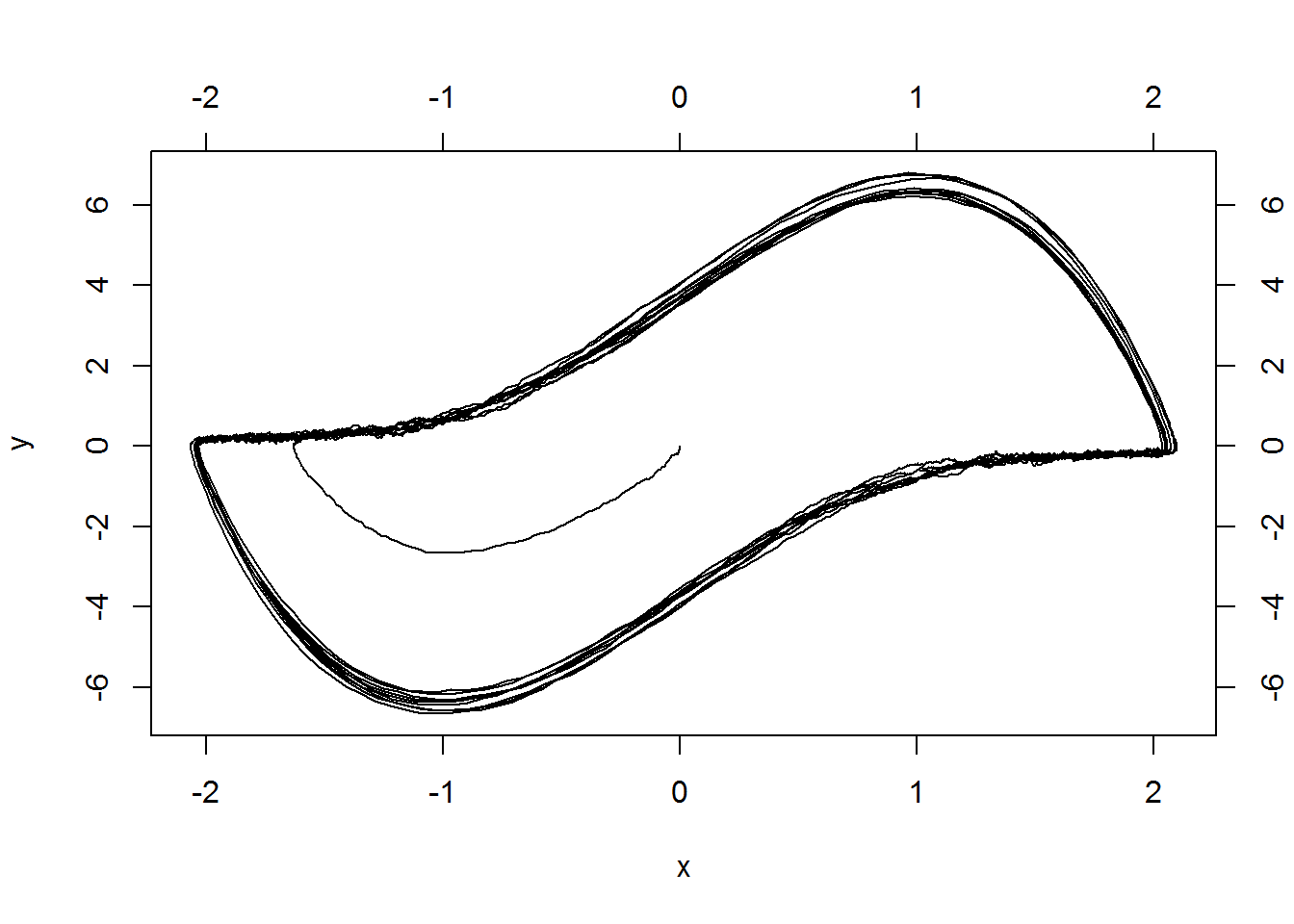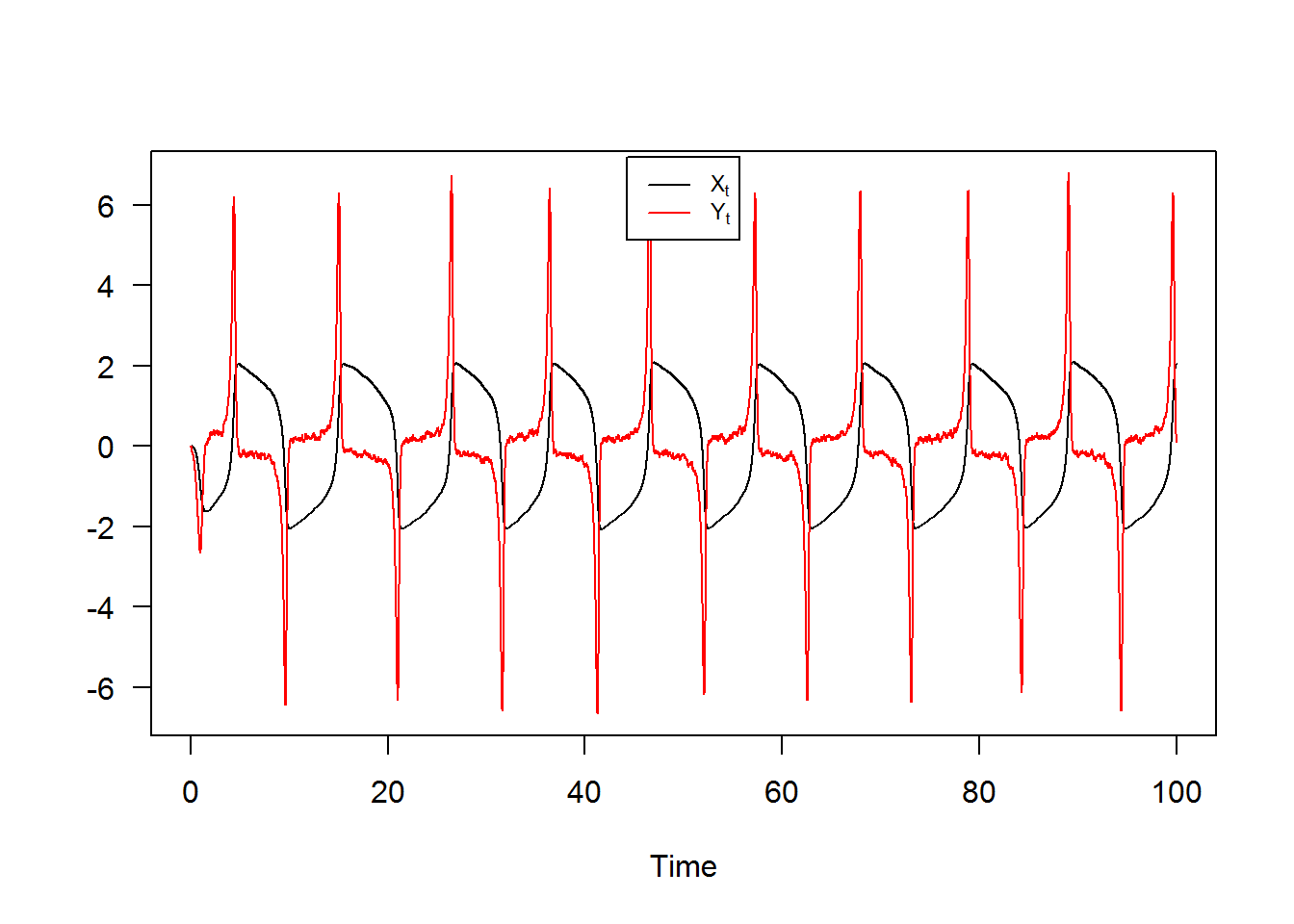# snssde3d()

The following $$3$$-dimensional SDE’s with a vector of drift and a diagonal matrix of diffusion coefficients:

Itô form: $\begin{equation}\label{eq17} \begin{cases} dX_t = f_{x}(t,X_{t},Y_{t},Z_{t}) dt + g_{x}(t,X_{t},Y_{t},Z_{t}) dW_{1,t}\\ dY_t = f_{y}(t,X_{t},Y_{t},Z_{t}) dt + g_{y}(t,X_{t},Y_{t},Z_{t}) dW_{2,t}\\ dZ_t = f_{z}(t,X_{t},Y_{t},Z_{t}) dt + g_{z}(t,X_{t},Y_{t},Z_{t}) dW_{3,t} \end{cases} \end{equation}$ Stratonovich form: $\begin{equation}\label{eq18} \begin{cases} dX_t = f_{x}(t,X_{t},Y_{t},Z_{t}) dt + g_{x}(t,X_{t},Y_{t},Z_{t}) \circ dW_{1,t}\\ dY_t = f_{y}(t,X_{t},Y_{t},Z_{t}) dt + g_{y}(t,X_{t},Y_{t},Z_{t}) \circ dW_{2,t}\\ dZ_t = f_{z}(t,X_{t},Y_{t},Z_{t}) dt + g_{z}(t,X_{t},Y_{t},Z_{t}) \circ dW_{3,t} \end{cases} \end{equation}$

$$W_{1,t}$$, $$W_{2,t}$$ and $$W_{3,t}$$ is a 3 independent standard Wiener process. To simulate this system using snssde3d() function we need to specify:

• The drift (3d) and diffusion (3d) coefficients as R expressions that depend on the state variables x, y , z and time variable t.
• The number of simulation steps N (default: N=1000).
• The number of the solution trajectories to be simulated by M (default: M=1).
• The initial conditions t0, x0 and end time T (default: t0=0, x0=c(0,0,0) and T=1).
• The integration step size Dt (default: Dt=(T-t0)/N).
• The choice of process types by the argument type="ito" for Ito or type="str" for Stratonovich (default type="ito").
• The numerical method to be used by method (default method="euler").

## Basic example

Assume that we want to describe the following SDE’s (3D) in Itô form: $\begin{equation}\label{eq0166} \begin{cases} dX_t = 4 (-1-X_{t}) Y_{t} dt + 0.2 dW_{1,t}\\ dY_t = 4 (1-Y_{t}) X_{t} dt + 0.2 dW_{2,t}\\ dZ_t = 4 (1-Z_{t}) Y_{t} dt + 0.2 dW_{3,t} \end{cases} \end{equation}$

We simulate a flow of $$10000$$ trajectories, with integration step size $$\Delta t = 0.001$$.

R> fx <- expression(4*(-1-x)*y , 4*(1-y)*x , 4*(1-z)*y)
R> gx <- rep(expression(0.2),3)
R> mod3d <- snssde3d(x0=c(x=2,y=-2,z=-2),drift=fx,diffusion=gx,M=1000)
R> mod3d
Itô Sde 3D:
| dX(t) = 4 * (-1 - X(t)) * Y(t) * dt + 0.2 * dW1(t)
| dY(t) = 4 * (1 - Y(t)) * X(t) * dt + 0.2 * dW2(t)
| dZ(t) = 4 * (1 - Z(t)) * Y(t) * dt + 0.2 * dW3(t)
Method:
| Euler scheme with order 0.5
Summary:
| Size of process   | N  = 1001.
| Number of simulation  | M  = 1000.
| Initial values    | (x0,y0,z0) = (2,-2,-2).
| Time of process   | t in [0,1].
| Discretization    | Dt = 0.001.

The following statistical measures (S3 method) for class snssde3d() can be approximated for the $$(X_{t},Y_{t},Z_{t})$$ process at any time $$t$$, for example at=1:

R> s = 1
R> mean(mod3d, at = s)
 -0.79985  0.87161  0.79477
R> moment(mod3d, at = s , center = TRUE , order = 2) ## variance
 0.0092235 0.0937483 0.0095991
R> Median(mod3d, at = s)
 -0.80874  0.84600  0.79681
R> Mode(mod3d, at = s)
 -0.81857  0.78889  0.78792
R> quantile(mod3d , at = s)
$x 0% 25% 50% 75% 100% -1.04829 -0.86966 -0.80874 -0.73775 -0.45068$y
0%      25%      50%      75%     100%
0.056109 0.670975 0.845998 1.058578 1.913511

$z 0% 25% 50% 75% 100% 0.44797 0.73410 0.79681 0.86127 1.05977  R> kurtosis(mod3d , at = s)  3.2379 3.2295 3.0485 R> skewness(mod3d , at = s)  0.41864 0.39115 -0.21822 R> cv(mod3d , at = s )  -0.12013 0.35146 0.12334 R> min(mod3d , at = s)  -1.048285 0.056109 0.447965 R> max(mod3d , at = s)  -0.45068 1.91351 1.05977 R> moment(mod3d, at = s , center= TRUE , order = 4)  0.00027601 0.02844016 0.00028146 R> moment(mod3d, at = s , center= FALSE , order = 4)  0.44379 1.07213 0.43500 The summary of the results of mod3d at time $$t=1$$ of class snssde3d() is given by: R> summary(mod3d, at = s)  Monte-Carlo Statistics for (X(t),Y(t),Z(t)) at time t = 1 X Y Z Mean -0.79985 0.87161 0.79477 Variance 0.00923 0.09384 0.00961 Median -0.80874 0.84600 0.79681 Mode -0.81857 0.78889 0.78792 First quartile -0.86966 0.67097 0.73410 Third quartile -0.73775 1.05858 0.86127 Minimum -1.04829 0.05611 0.44797 Maximum -0.45068 1.91351 1.05977 Skewness 0.41864 0.39115 -0.21822 Kurtosis 3.23793 3.22951 3.04848 Coef-variation -0.12013 0.35146 0.12334 3th-order moment 0.00037 0.01124 -0.00021 4th-order moment 0.00028 0.02844 0.00028 5th-order moment 0.00003 0.00961 -0.00002 6th-order moment 0.00002 0.01469 0.00001 For plotting (back in time) using the command plot, and plot3D in space the results of the simulation are shown in Figure 9. R> plot(mod3d,union = TRUE) ## back in time R> plot3D(mod3d,display="persp") ## in space (O,X,Y,Z)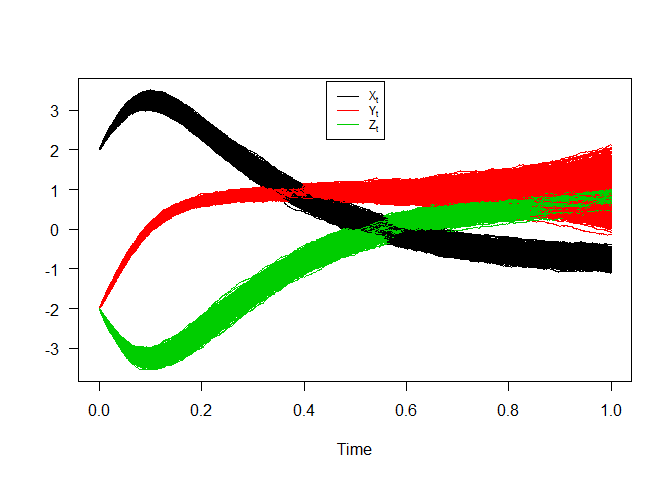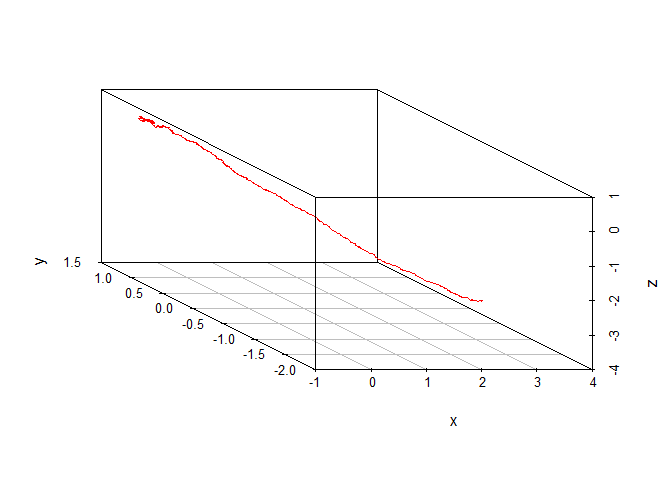3D SDEs Hence we can just make use of the rsde3d() function to build our random number for $$(X_{t},Y_{t},Z_{t})$$ at time $$t = 1$$. R> out <- rsde3d(object = mod3d, at = s) R> head(out,n=10)  x y z 1 -0.72964 0.74243 0.85396 2 -0.68972 0.72162 0.62938 3 -0.83193 0.57660 0.83239 4 -0.75814 0.59987 0.65566 5 -0.82004 0.76703 0.81252 6 -0.67802 0.71623 0.90862 7 -0.75356 0.54518 0.58515 8 -0.76021 1.09544 0.78467 9 -0.73605 0.58832 0.68057 10 -0.94260 0.91681 0.70699 R> summary(out)  x y z Min. :-1.048 Min. :0.0561 Min. :0.448 1st Qu.:-0.870 1st Qu.:0.6710 1st Qu.:0.734 Median :-0.809 Median :0.8460 Median :0.797 Mean :-0.800 Mean :0.8716 Mean :0.795 3rd Qu.:-0.738 3rd Qu.:1.0586 3rd Qu.:0.861 Max. :-0.451 Max. :1.9135 Max. :1.060  R> cov(out)  x y z x 0.0092327 -0.015766 -0.0037222 y -0.0157659 0.093842 0.0163150 z -0.0037222 0.016315 0.0096087 For each SDE type and for each numerical scheme, the marginal density of $$X_t$$, $$Y_t$$ and $$Z_t$$ at time $$t=1$$ are reported using dsde3d() function, see e.g. Figure 10. R> den <- dsde3d(mod3d,pdf="M",at =1) R> den  Marginal density of X(t-t0)|X(t0)=2 at time t = 1 Data: x (1000 obs.); Bandwidth 'bw' = 0.02172 x f(x) Min. :-1.11345 Min. :0.0002 1st Qu.:-0.93147 1st Qu.:0.1054 Median :-0.74948 Median :0.6633 Mean :-0.74948 Mean :1.3724 3rd Qu.:-0.56750 3rd Qu.:2.7325 Max. :-0.38551 Max. :4.3231 Marginal density of Y(t-t0)|Y(t0)=-2 at time t = 1 Data: y (1000 obs.); Bandwidth 'bw' = 0.06539 y f(y) Min. :-0.14007 Min. :0.00010 1st Qu.: 0.42237 1st Qu.:0.04042 Median : 0.98481 Median :0.24352 Mean : 0.98481 Mean :0.44406 3rd Qu.: 1.54725 3rd Qu.:0.81514 Max. : 2.10969 Max. :1.38751 Marginal density of Z(t-t0)|Z(t0)=-2 at time t = 1 Data: z (1000 obs.); Bandwidth 'bw' = 0.02146 z f(z) Min. :0.38360 Min. :0.0002 1st Qu.:0.56873 1st Qu.:0.0917 Median :0.75387 Median :0.7179 Mean :0.75387 Mean :1.3491 3rd Qu.:0.93900 3rd Qu.:2.5052 Max. :1.12413 Max. :4.0333  R> plot(den, main="Marginal Density")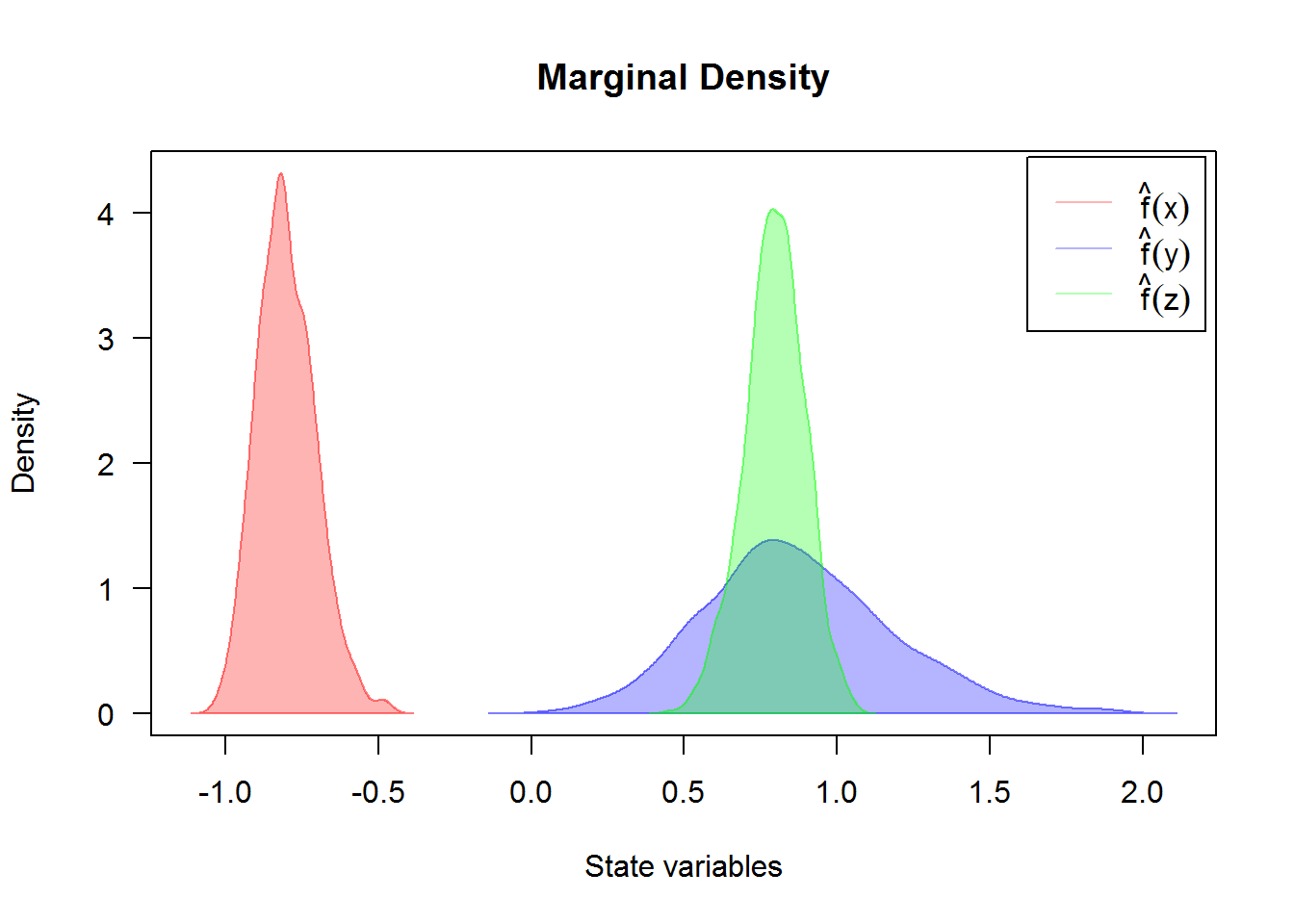For an approximate joint transition density for $$(X_t,Y_t,Z_t)$$ (for more details, see package sm or ks.) R> denJ <- dsde3d(mod3d,pdf="J") R> plot(denJ,display="rgl") Return to snssde3d() ## Attractive model for 3D diffusion processes If we assume that $$U_w( x , y , z , t )$$, $$V_w( x , y , z , t )$$ and $$S_w( x , y , z , t )$$ are neglected and the dispersion coefficient $$D( x , y , z )$$ is constant. A system becomes (see Boukhetala,1996): $\begin{eqnarray}\label{eq19} % \nonumber to remove numbering (before each equation) \begin{cases} dX_t = \left(\frac{-K X_{t}}{\sqrt{X^{2}_{t} + Y^{2}_{t} + Z^{2}_{t}}}\right) dt + \sigma dW_{1,t} \nonumber\\ dY_t = \left(\frac{-K Y_{t}}{\sqrt{X^{2}_{t} + Y^{2}_{t} + Z^{2}_{t}}}\right) dt + \sigma dW_{2,t} \\ dZ_t = \left(\frac{-K Z_{t}}{\sqrt{X^{2}_{t} + Y^{2}_{t} + Z^{2}_{t}}}\right) dt + \sigma dW_{3,t} \nonumber \end{cases} \end{eqnarray}$ with initial conditions $$(X_{0},Y_{0},Z_{0})=(1,1,1)$$, by specifying the drift and diffusion coefficients of three processes $$X_{t}$$, $$Y_{t}$$ and $$Z_{t}$$ as R expressions which depends on the three state variables (x,y,z) and time variable t, with integration step size Dt=0.0001. R> K = 4; s = 1; sigma = 0.2 R> fx <- expression( (-K*x/sqrt(x^2+y^2+z^2)) , (-K*y/sqrt(x^2+y^2+z^2)) , (-K*z/sqrt(x^2+y^2+z^2)) ) R> gx <- rep(expression(sigma),3) R> mod3d <- snssde3d(drift=fx,diffusion=gx,N=10000,x0=c(x=1,y=1,z=1)) R> mod3d Itô Sde 3D: | dX(t) = (-K * X(t)/sqrt(X(t)^2 + Y(t)^2 + Z(t)^2)) * dt + sigma * dW1(t) | dY(t) = (-K * Y(t)/sqrt(X(t)^2 + Y(t)^2 + Z(t)^2)) * dt + sigma * dW2(t) | dZ(t) = (-K * Z(t)/sqrt(X(t)^2 + Y(t)^2 + Z(t)^2)) * dt + sigma * dW3(t) Method: | Euler scheme with order 0.5 Summary: | Size of process | N = 10001. | Number of simulation | M = 1. | Initial values | (x0,y0,z0) = (1,1,1). | Time of process | t in [0,1]. | Discretization | Dt = 0.0001. The results of simulation are shown: R> plot3D(mod3d,display="persp",col="blue")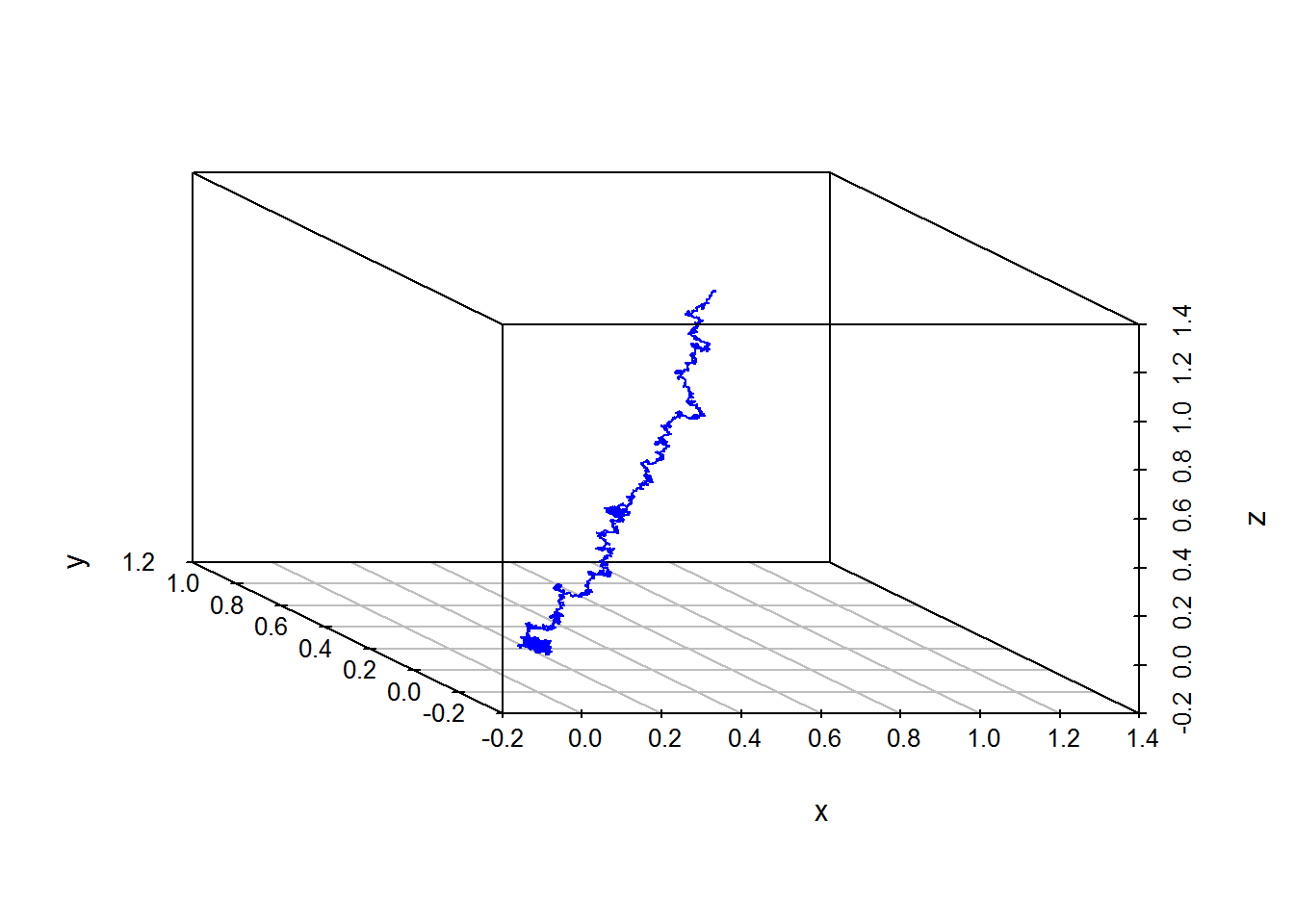Return to snssde3d() ## Transformation of an SDE one-dimensional Next is an example of one-dimensional SDE driven by three independent Brownian motions ($$W_{1,t}$$,$$W_{2,t}$$,$$W_{3,t}$$), as follows: $\begin{equation}\label{eq20} dX_{t} = \mu W_{1,t} dt + \sigma W_{2,t} \circ dW_{3,t} \end{equation}$ To simulate the solution of the process $$X_t$$, we make a transformation to a system of three equations as follows: $\begin{eqnarray}\label{eq21} \begin{cases} % \nonumber to remove numbering (before each equation) dX_t = \mu Y_{t} dt + \sigma Z_{t} \circ dW_{3,t} \nonumber\\ dY_t = dW_{1,t} \\ dZ_t = dW_{2,t} \nonumber \end{cases} \end{eqnarray}$ run by calling the function snssde3d() to produce a simulation of the solution, with $$\mu = 1$$ and $$\sigma = 1$$. R> fx <- expression(y,0,0) R> gx <- expression(z,1,1) R> modtra <- snssde3d(drift=fx,diffusion=gx,M=1000,type="str") R> modtra Stratonovich Sde 3D: | dX(t) = Y(t) * dt + Z(t) o dW1(t) | dY(t) = 0 * dt + 1 o dW2(t) | dZ(t) = 0 * dt + 1 o dW3(t) Method: | Euler scheme with order 0.5 Summary: | Size of process | N = 1001. | Number of simulation | M = 1000. | Initial values | (x0,y0,z0) = (0,0,0). | Time of process | t in [0,1]. | Discretization | Dt = 0.001. R> summary(modtra)  Monte-Carlo Statistics for (X(t),Y(t),Z(t)) at time t = 1 X Y Z Mean -0.03370 -0.06637 -0.01571 Variance 0.80281 0.96396 0.96160 Median -0.05583 -0.03795 -0.02365 Mode -0.14771 0.12565 -0.07586 First quartile -0.52031 -0.72023 -0.69051 Third quartile 0.45321 0.57364 0.63421 Minimum -4.06546 -3.78095 -3.21498 Maximum 5.73258 3.03095 3.55463 Skewness 0.31378 -0.10885 0.01810 Kurtosis 6.28872 3.23703 3.16024 Coef-variation -26.58940 -14.79238 -62.40626 3th-order moment 0.22570 -0.10302 0.01707 4th-order moment 4.05308 3.00792 2.92220 5th-order moment 7.94984 -1.44217 0.11985 6th-order moment 65.53119 16.71270 15.28423 the following code produces the result in Figure 12. R> plot(modtra$X,plot.type="single",ylab=expression(X[t]))
R> lines(time(modtra),apply(modtra$X,1,mean),col=2,lwd=2) R> legend("topleft",c("mean path"),col=2,lwd=2,cex=0.8)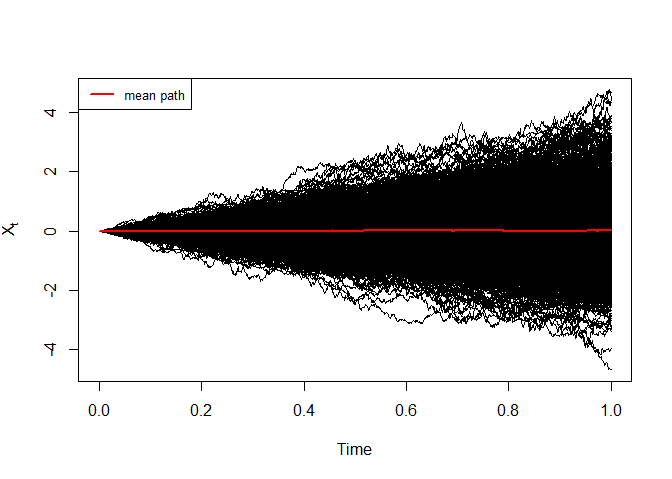Simulation of $$X_t$$ The histogram and kernel density of $$X_t$$ at time $$t=1$$ are reported using dsde3d() function, see e.g. Figure 13. R> den <- dsde3d(modtra,pdf="Marginal",at=1) R> den$resx

Call:
density.default(x = x, na.rm = TRUE)

Data: x (1000 obs.);    Bandwidth 'bw' = 0.1642

x                y
Min.   :-4.558   Min.   :0.00003
1st Qu.:-1.862   1st Qu.:0.00176
Median : 0.834   Median :0.00435
Mean   : 0.834   Mean   :0.09264
3rd Qu.: 3.529   3rd Qu.:0.11711
Max.   : 6.225   Max.   :0.55426  
R> MASS::truehist(den$ech$x,xlab = expression(X[t==1]));box()
R> lines(den$resx,col="red",lwd=2) R> legend("topleft",c("Distribution histogram","Kernel Density"),inset =.01,pch=c(15,NA),lty=c(NA,1),col=c("cyan","red"), lwd=2,cex=0.8)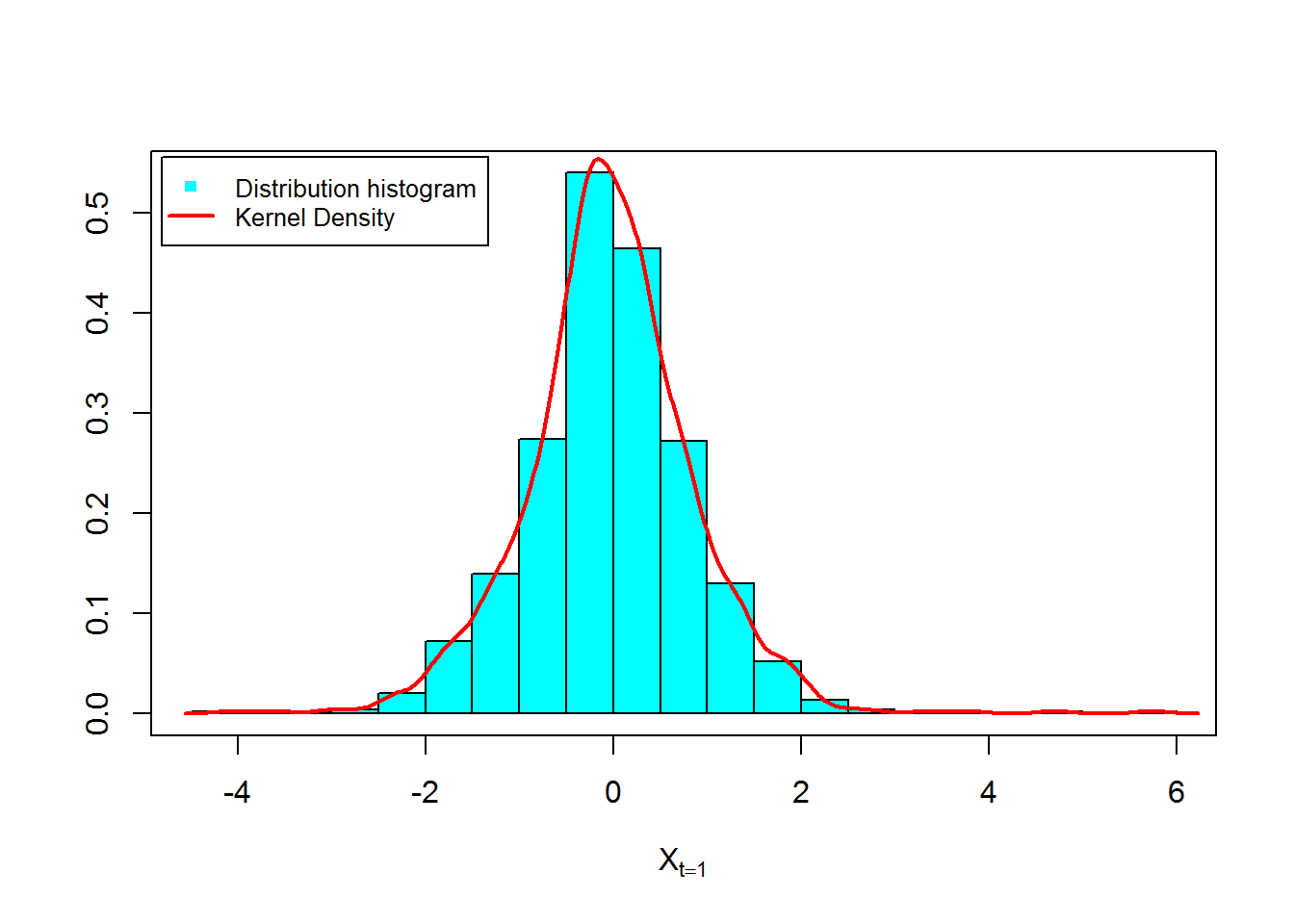Figure 14 and 15, show approximation results for $$m_{1}(t)= \text{E}(X_{t})$$, $$S_{1}(t)=\text{V}(X_{t})$$ and $$C(s,t)=\text{Cov}(X_{s},X_{t})$$: R> m1 <- apply(modtra$X,1,mean) ## m1(t)
R> S1  <- apply(modtra$X,1,var) ## s1(t) R> out_a <- data.frame(m1,S1) R> matplot(time(modtra), out_a, type = "l", xlab = "time", ylab = "", col=2:3,lwd=2,lty=2:3,las=1) R> legend("topleft",c(expression(m(t),S(t))),inset = .09,col=2:3,lty=2:3,lwd=2,cex=0.9)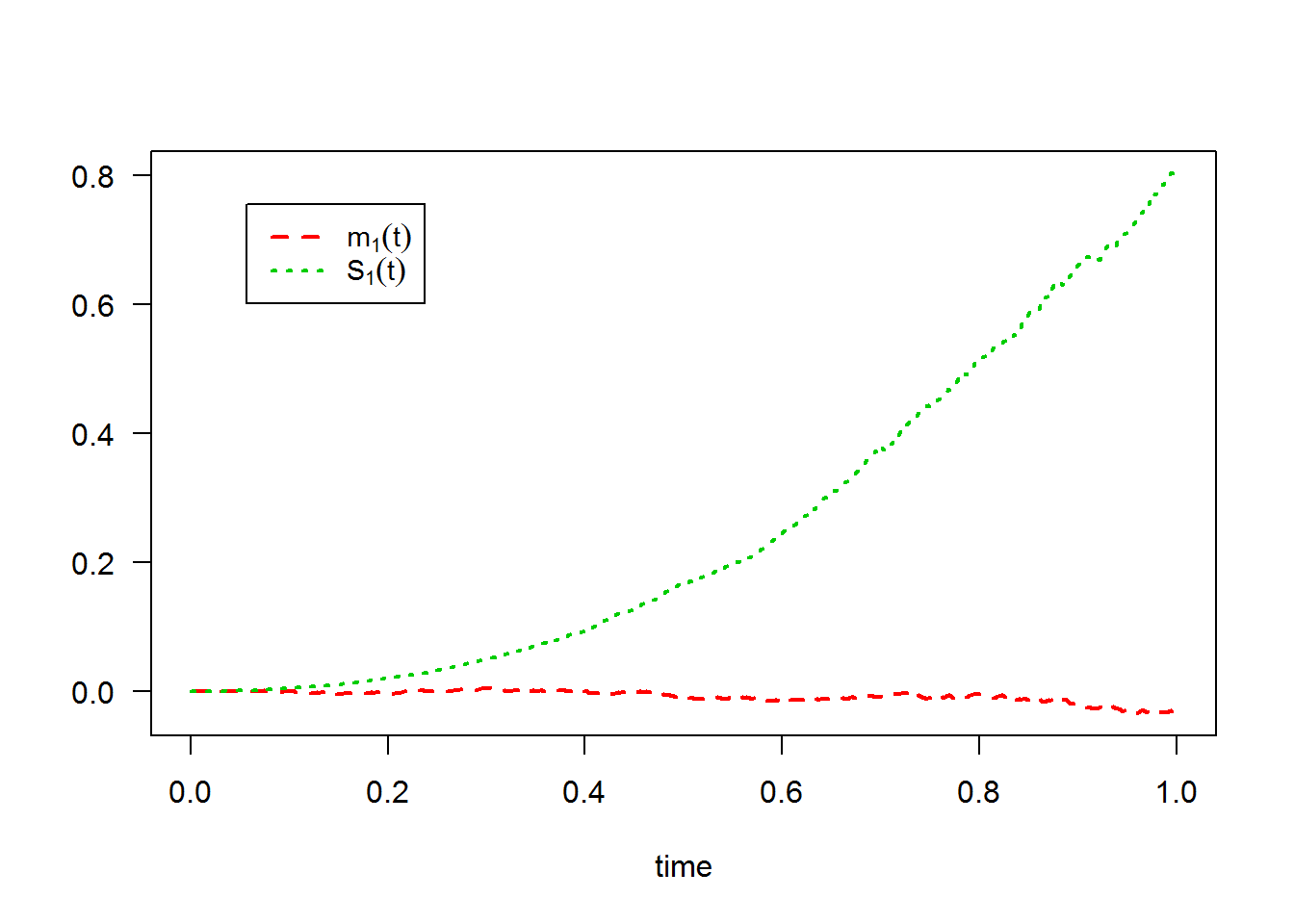R> color.palette=colorRampPalette(c('white','green','blue','red')) R> filled.contour(time(modtra), time(modtra), cov(t(modtra$X)), color.palette=color.palette,plot.title = title(main = expression(paste("Covariance empirique:",cov(X[s],X[t]))),xlab = "time", ylab = "time"),key.title = title(main = ""))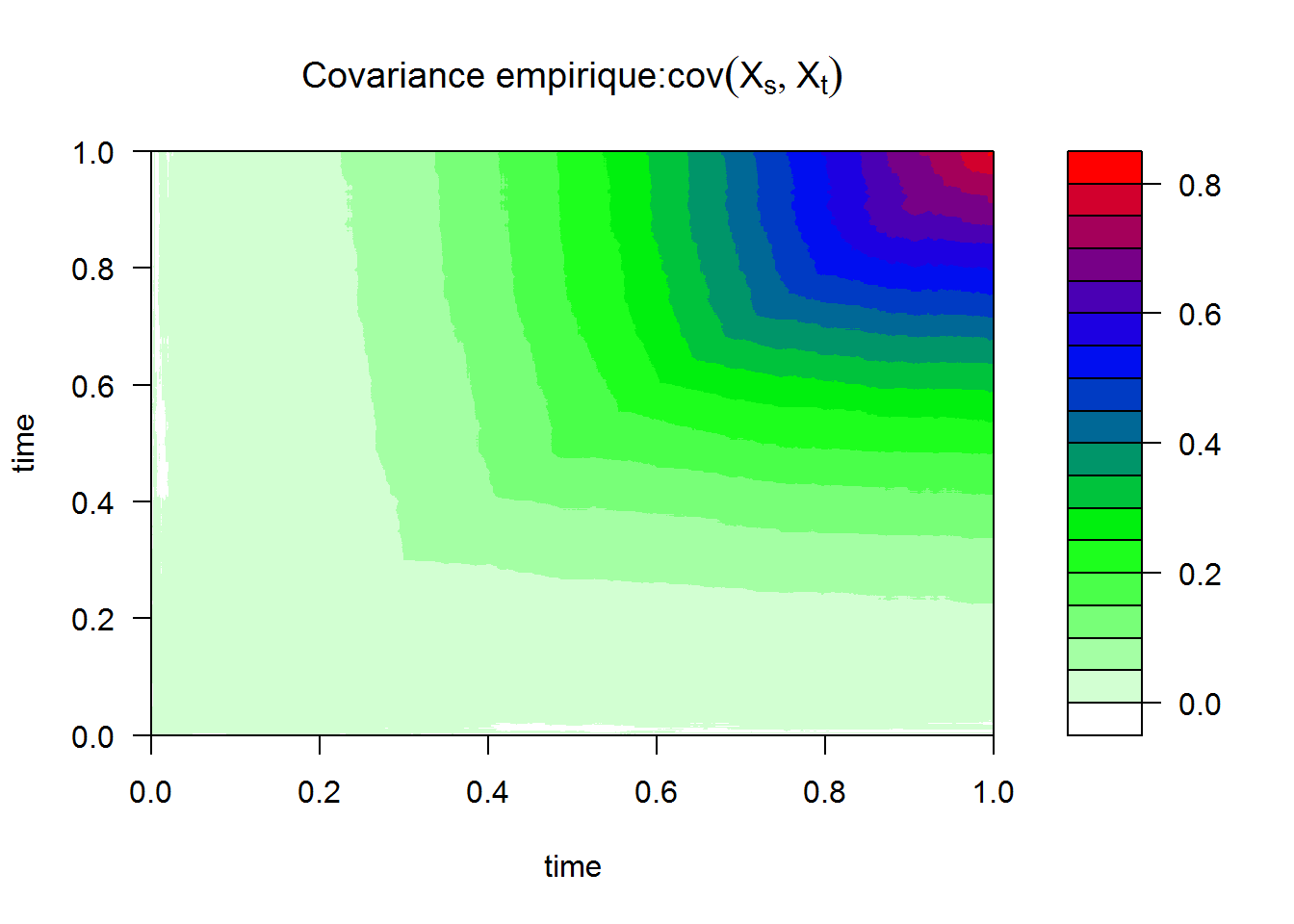3. The equivalently of $$X_{t}^{\text{mod1}}$$ the following Stratonovich SDE: $$dX_{t} = \theta X_{t} \circ dW_{t}$$.